# Coding-Decoding

Coding means to hide the meaning of any message and decoding means to understand the actual meaning of that message.

In questions based on coding-decoding, a word or sentence is coded in a particular way and the candidates are asked to code other word or sentence in the same way or to decode the word or sentence.

To solve these questions, the candidates are required to understand the rule that has been followed to code a particular word/message.

## Position of Letters in English Alphabet

Sometimes a word or a sentence is coded by changing the position of English alphabet letters according to a definite pattern, so it is necessary to remember the positions of all the letters in English alphabetical order, both in forward and backward order.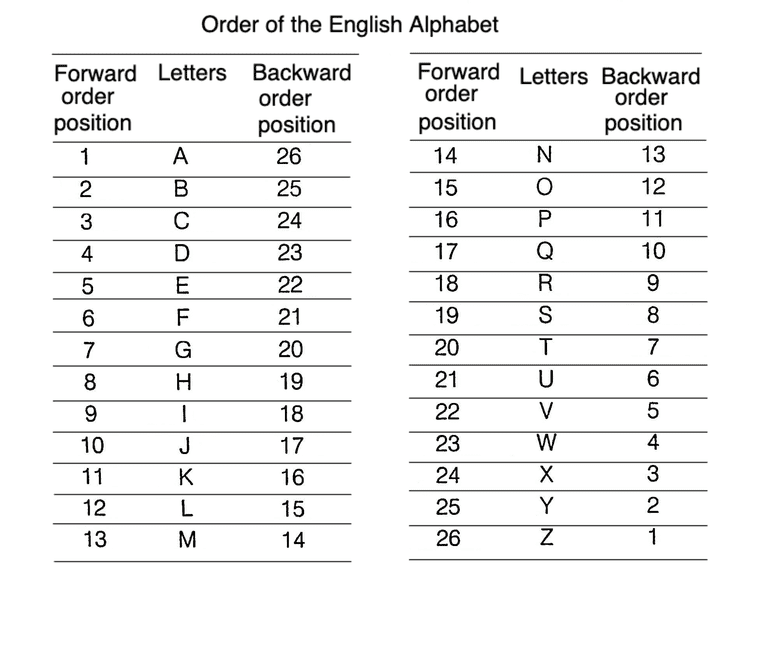## Circular Arrangement of Letters in English Alphabet

1: Clockwise Arrangement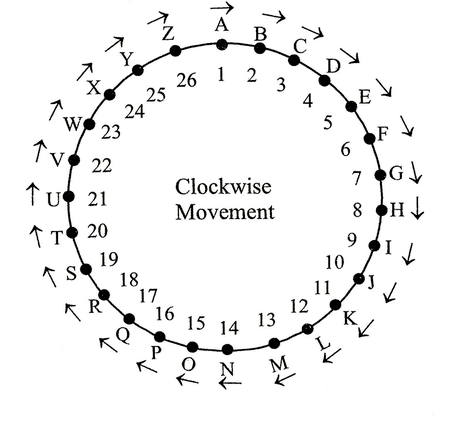2: Anti-clockwise Arrangement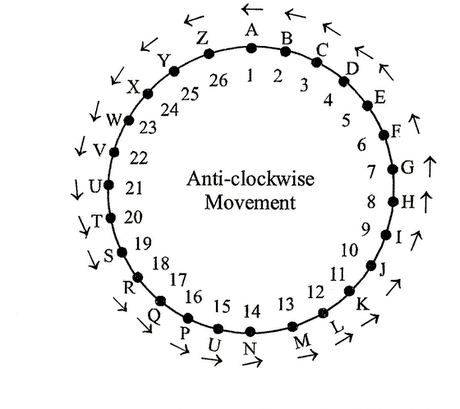If we have to find the letter one placed forward to A, then the letter will be B and this result can be found out by using either linear or circular arrangement but when one is asked to find the letter which is one letter backward of A (Z is one letter backward A) or one letter forward of Z (A is the one letter forward Z), then only circular arrangement gives such results.

## Method to Remember the Position of Letters in English Alphabet

Some method/techniques are given to remember the positions of English alphabets in forward or backward order.

1. By using EJOTY and CFILORUX formulae, we can easily remember the position of letters of English alphabet in forward order.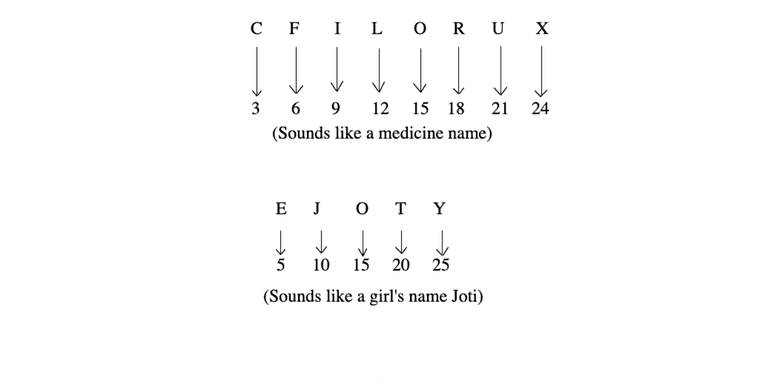Note:

Backward order position of a letter
= 27 - Forward order position of letter

e.g. Backward order position of B

= 27 - Forward order position of B
= 27 - 2 = 25

2. By Using V Q L G B and X U R O L I F C, we can easily remember the position of letters of English alphabet is reverse order.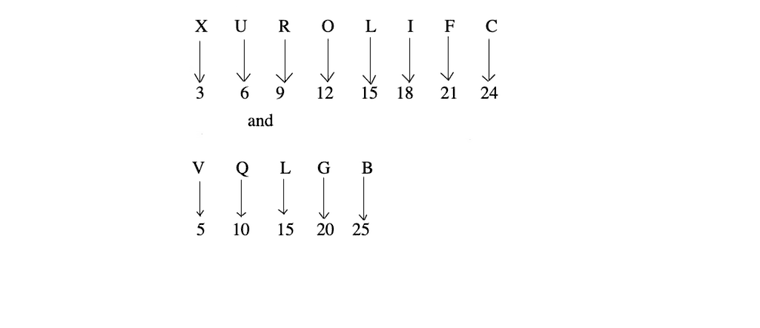## Types of Coding - Decoding

Following are the categories/Types of coding-decoding, which are generally asked in various competitive examinations.

Type #1: Letter/Number Coding

In this type of coding, we deal with questions, in which the letters of a word are replaced by certain other letters or numbers according to a specific pattern/rule to form a code. You are required to detect the coding pattern/rule and answer the question(s) that are asked, based on that coding pattern/rule.

Example 1: In a certain code language, 'GIVE' is written as 'VIEG' and 'OVER' is written as "EVRO'. How will 'DISK' be written in that same code?

(a) SIDK

(b) KISD

(c) KDSI

(d) SIKD

(e) None of these

Solution:  (d) Here,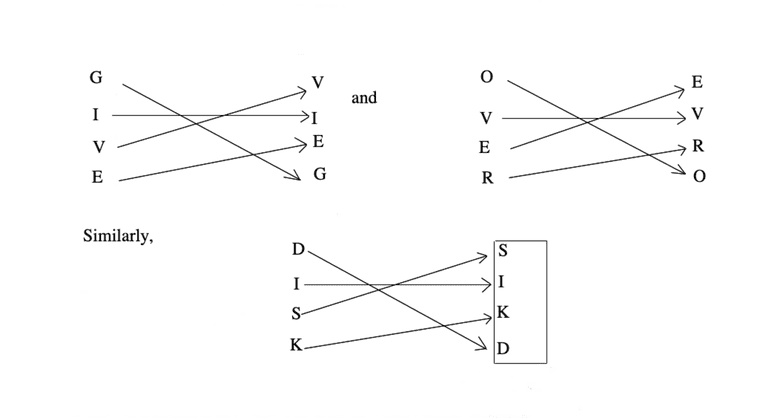Example 2: If in a certain code 'INTELLIGENCE' is written as 'ETNIGILLECNE', then how can 'MATHEMATICAL' be written in the same code?

(a) AMHTMETACILA           (b) TAMMEHTALAC

(c) HTAMTAMELACI            (d) LACITAMEHTAM

Solution:  (c) As,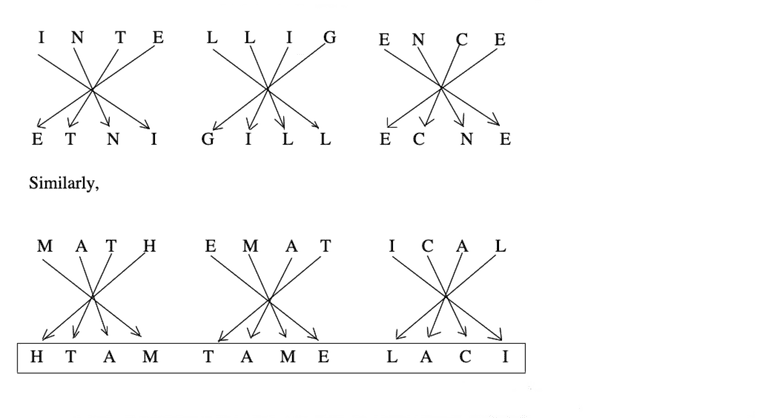Example 3: If GOODNESS is coded as HNPCODTR, then how GREATNESS can be written in that code?

(a) HQZSMFRT

(b) HQFZUFRTM

(c) HQFZUODTR

(d) HQFZUMFRT

Solution:  (d) Clearly, the letters in the word GOODNESS are moved one step forward and one step backward, alternately to obtain the letters of the code.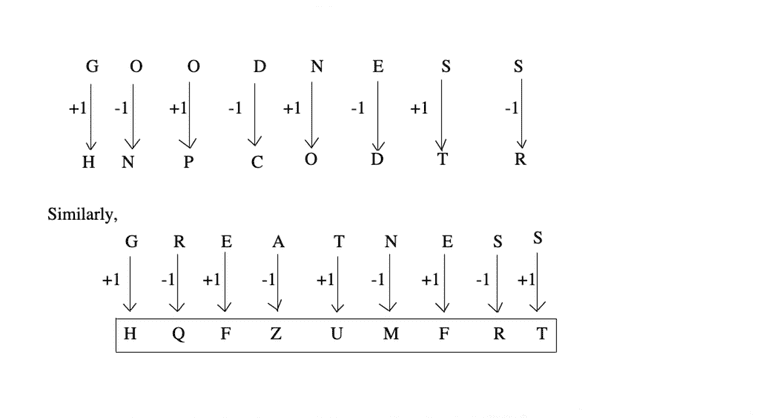Example 4: If GRASP is code as TIZHK, what will be code as OVTZXB?

(a) LEGATE            (b) LEAGUE

(c) LEGACY            (d) LEDGER

Solution:  (c) As,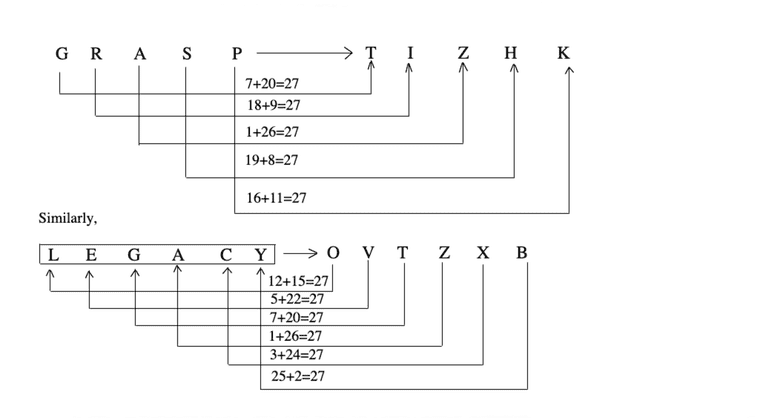Example 5: If 'WORK' is coded as '4-12-9-16', then how will you code 'WOMAN'?

(a) 4-12-14-26-13         (b) 4-26-14-13-12

(c) 23-12-26-14-13       (d) 23-15-13-1-14

Solution:  (a) We have,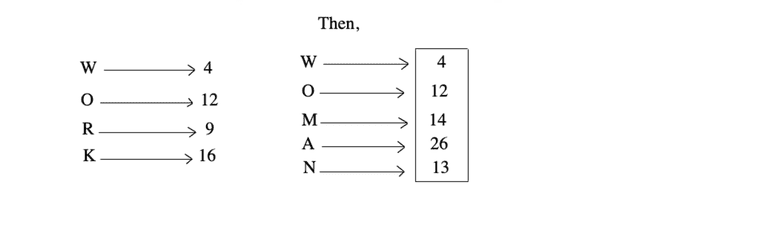Here, each letter is coded by the numerical value obtained by subtracting its 'position value' in English alphabetical order, from 27, e.g. letters W, O, M, A, N are at 23rd, 15th, 13th, 1st and 14th position in alphabetical order. So, their codes are (27 - 23), (27 - 15), (27 - 13), (27 - 1), (27 - 14), i.e. 4, 12, 14, 26, 13 respectively i.e. their backward order position.

Example 6: If CUP = 40, then KITE = ?

(a) 10              (b) 20

(c) 30              (d) 45

Solution:  (d) Using forward letter positions,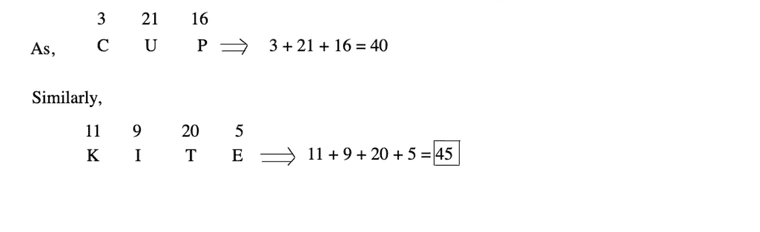Example 7: If BAG = 71, then VICE = ?

(a) 69               (b) 70

(c) 75               (d) 90

Solution:  (a) Using backward letter positions,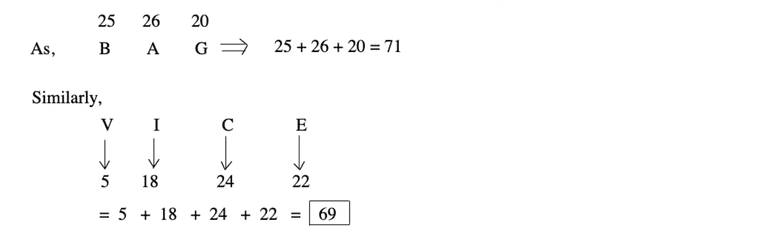Type #2: Direct Coding

In direct coding, the code letters/numbers/ symbols occur in the same sequence as the corresponding letters occur in the words. This is basically a direct substitution method.

Example 8: If DEVICE is coded as BAREXA and ORNATE is coded as ZVMESA, what VIDEO be coded as?

(a) RIBAZ          (b) REBAZ

(c) RBEAZ         (d) ZABER

Solution:  (d) Using direct letter coding method,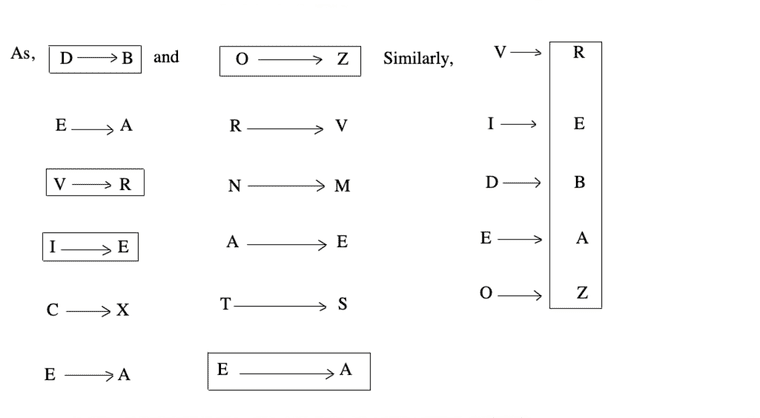Example 9: In a certain code language, FILE is written as 7465 and IDEAL is written as 43586. How will DEAF be written in that code language?

(a) 3478            (b) 3588

(c) 3587            (d) 4578

Solution:  (d) Using direct number coding method,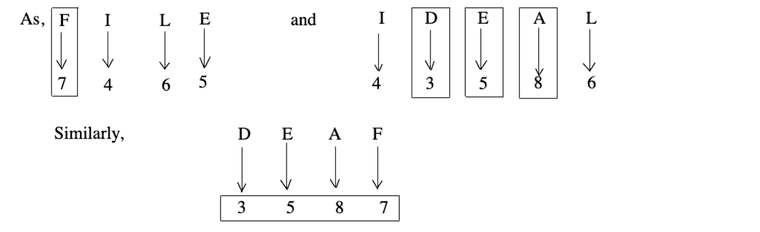Example 9: In a certain code, 'GONE' is written as 5 % 2 # and 'MEDAL' is written as 4 # 3 \$ @, then how will 'GOLD' be written in that code?

(a) 5 @ % 3

(b) 5 % @ 3

(c) 5 # @ 3

(d) 5 % # 3

Solution:  (b) Using direct coding method,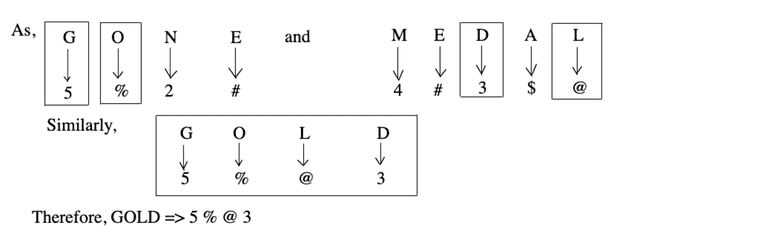Type #3: Conditional Coding

In this type, letters/numbers are given along with their code. Candidates are required to find the codes for a particular letter group/word/number according to certain conditions given in the questions.

Directions (Examples 11 - 13) Study the following letters and their corresponding digits code followed by certain conditions of coding and then answer the questions given below them by finding out which of the digits combinations given in (a), (b), (c) and (d) is the coded form of the letters-groups given in each question and mark your answer accordingly.

 Letters Digits/Codes P N A J I R E B U K 5 3 9 1 4 6 2 7 0 8

Conditions

(I)    If both the first and the last letters in the group are vowels, both should be coded as \$.

(II)   If both the first and the last letters in the group are consonants, both should be coded as #.

Example 11: KUNAJB

(a) 803917

(b) \$0391\$

(c) #0391#

(d) #0391\$

(e) None of these

Solution:  (c) We know that, in English alphabets A, E, I, O, U letters are vowels and remaining letters are consonants.

From condition (II),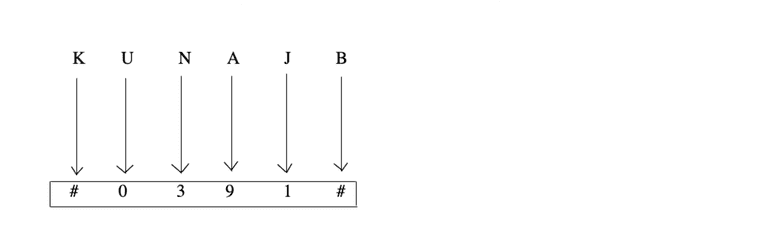Example 12: RBUKAE

(a) #70892

(b) 670892

(c) 670982

(d) 607892

(d) None of these

Solution:  (b) We know that, in English alphabets A, E, I, O, U letters are vowels and remaining letters are consonants.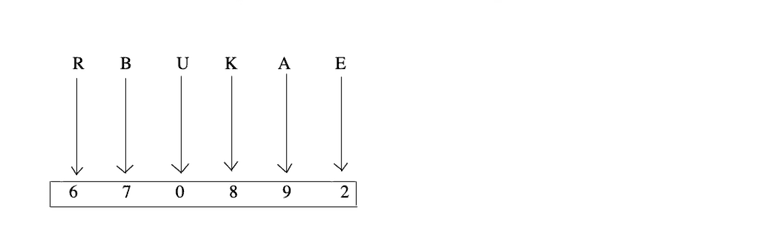Note This question does not follow any condition.

Example 13: EBNAPI

(a) 273954

(b) \$7395\$

(c) #7395#

(d) \$7395#

(e) None of these

Solution:  (b) We know that, in English alphabets A, E, I, O, U letters are vowels and remaining letters are consonants.

From condition (I),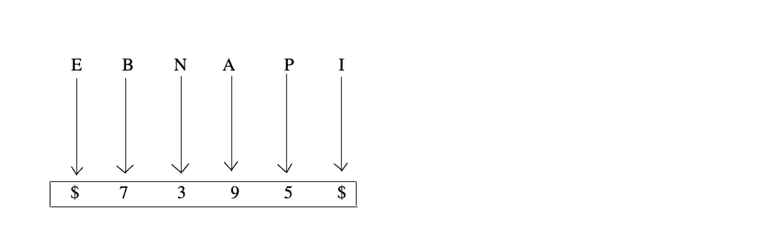Type #4: Fictitious Language Coding

Here, coded form of two or more sentences is given and you are required to find the code of a particular word or message.

To analyse such codes, any two messages bearing a common word/number are picked up. The common code will represent that particular word/number.

Proceeding similarly by picking up all possible combinations of two, the entire message can be decoded and the codes for every individual word/number can be found.

In some cases, there is no common word/number in the message given. In those cases, the words/numbers are coded separately. You have to identify the correct pattern of coding and find the code for the word or message given in the question.

Example 14: In a certain code language, 'it pit sit' means 'I am boy', 'it nit sit' means 'I am girl', which of the following means 'girl'?

(a) it

(b) pit

(c) sit

(d) nit

(e) None of these

Solution:  (d) according to the question, we have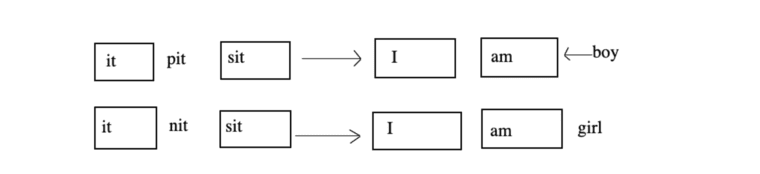Here, 'it' and 'sit' are common in both the codes and 'I' and 'am' are common in both messages.

Hence, code for girl will br 'nit'.

Example 15: In a certain code language, 481 means 'sky is blue', 246 means 'sea is deep' and 698 means 'sea looks blue'. What number is the code for 'blue'?

(a) 1     (b) 6      (c) 8      (d) 9

Solution:  (c) according to the question, we have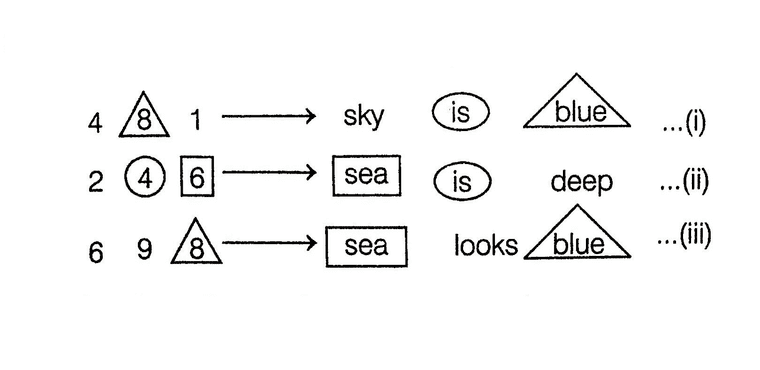From Eqs. (i) and (ii), we see that the code for blue is '8'.

Directions (Examples 16 - 17) Study the following information carefully and answer the questions given below.

In a certain code language
'given time simple plan' is written as
'@E4 & N4 % N5 # E6'
'tried solution plant great' is written as
'#N8 @ D5 % T5 & T5'
'sick point good turn' is written as
'#k4 % D4 @ N4 & T5'
'garden sister phone team' os written as
'&E5 # R6 % N6 @ M4'

Example 16: Which of the following is the code for 'translate'?

(a) @E84

(b) @E9

(c) #E8

(d) #T8

(e) #T9

Solution:  (b) Here, symbols are coded as per the first letter of the word.

As for 'g' symbol is %.

similarly, 'p' ---> &, 't' ---->@ and 's' ----> #

The number in the code represents the number of letter in the word and the letter in the code represents the last letter of the word.

e.g. In the word "given" g = %, last letter = N and number of letters = 5

Therefore, Given = % N5

Code for translate -----> @E9

Example 17: In the given code language, what does the code '%D4' represent?

(a) Point

(b) Turn

(c) Sick

(d) Good

(e) None of these

Solution:  (d) Here, symbols are coded as per the first letter of the word.

As for 'g' symbol is %.

similarly, 'p' ---> &, 't' ---->@ and 's' ----> #

The number in the code represents the number of letter in the word and the letter in the code represents the last letter of the word.

e.g. In the word "given" g = %, last letter = N and number of letters = 5

Therefore, Given = % N5

% -----> g

So, from the options %D4 ----> Good

Type #5: Substitution Coding

In this type of coding, some particular words are coded with certain substituted word and on the basis of substituted word, the code of given word is derived.

Example 18: If 'white' is called 'blue', 'blue' is called 'red', 'red' is called 'yellow', 'yellow, is called 'green', 'green' is called 'black', 'black' is called 'violet' and 'violet' is called 'orange', then what would be the colour of human blood?

(a) Red

(b) Green

(c) Yellow

(d) Violet

(e) Orange

Solution:  (c) We know that, the colour of human blood is 'red' but here 'red' is called 'yellow'. So, the colour of human blood will be 'yellow'.

Example 19: If 'parrot' is known as 'peacock', 'peacock is known as 'swallow', 'swallow' is known as 'pigeon' and 'pigeon' is known as 'sparrow', then what would be the name of Indian National Bird?

(a) Parrot

(b) Swallow

(c) Peacock

(d) Pigeon

Solution:  (b) We know that, peacock is the Indian National Bird but here peacock is known as swallow.

Example 20: On another planet the local terminology for 'earth', 'water', 'light' 'air' and 'sky' are sky, light, 'air', water and 'earth' respectively. If someone is thirsty there what would he drink?

(a) Light

(b) Air

(c) Sky

(d) Water

Solution:  (a) One drinks water when he is thirsty.

since, 'water' drink 'light' when he is thirsty there. So, the answer is 'light'.

LearnFrenzy provides you lots of fully solved "Coding Decoding" Questions and Answers with explanation.